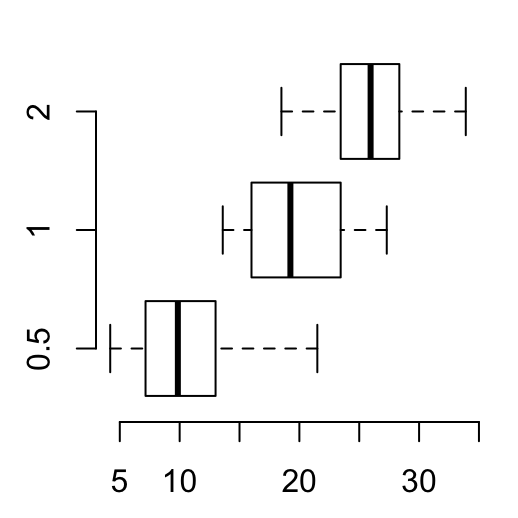# Box Plots - R Base Graphs

Previously, we described the essentials of R programming and provided quick start guides for importing data into R.

Here, we’ll describe how to create box plots in R.

1. Launch RStudio as described here: Running RStudio and setting up your working directory

2. Prepare your data as described here: Best practices for preparing your data and save it in an external .txt tab or .csv files

3. Import your data into R as described here: Fast reading of data from txt|csv files into R: readr package.

Here, we’ll use the R built-in ToothGrowth data set.

``````# Print the first 6 rows
``````##    len supp dose
## 1  4.2   VC  0.5
## 2 11.5   VC  0.5
## 3  7.3   VC  0.5
## 4  5.8   VC  0.5
## 5  6.4   VC  0.5
## 6 10.0   VC  0.5``````

# R base box plots: boxplot()

Draw a box plot of teeth length (len):

• Basic box plots
``````# Box plot of one variable
boxplot(ToothGrowth\$len)
# Box plots by groups (dose)
# remove frame
boxplot(len ~ dose, data = ToothGrowth, frame = FALSE)
# Horizontal box plots
boxplot(len ~ dose, data = ToothGrowth, frame = FALSE,
horizontal = TRUE)
# Notched box plots
boxplot(len ~ dose, data = ToothGrowth, frame = FALSE,
notch = TRUE)``````Notch is used to compare groups. In the notched boxplot, if two boxes’ notches do not overlap this is “strong evidence” their medians differ (Chambers et al., 1983, p. 62).

• Change group names
``````boxplot(len ~ dose, data = ToothGrowth, frame = FALSE,
names = c("D0.5", "D1", "D2"))``````• Change color
``````# Change the color of border using one single color
boxplot(len ~ dose, data = ToothGrowth, frame = FALSE,
border = "steelblue")
# Change the color of border.
#  Use different colors for each group
boxplot(len ~ dose, data = ToothGrowth, frame = FALSE,
border = c("#999999", "#E69F00", "#56B4E9"))
# Change fill color : single color
boxplot(len ~ dose, data = ToothGrowth, frame = FALSE,
col = "steelblue")
# Change fill color: multiple colors
boxplot(len ~ dose, data = ToothGrowth, frame = FALSE,
col = c("#999999", "#E69F00", "#56B4E9"))``````• Box plot with multiple groups
``````boxplot(len ~ supp*dose, data = ToothGrowth,
col = c("white", "steelblue"), frame = FALSE)``````• Change main title and axis labels
``````# Change axis titles
# Change color (col = "gray") and remove frame
# Create notched box plot
boxplot(len ~ dose, data = ToothGrowth,
main = "Plot of length by dose",
xlab = "Dose (mg)", ylab = "Length",
col = "lightgray", frame = FALSE)``````# Box plot with the number of observations: gplots::boxplot2()

The function boxplot2()[in gplots package] can be used to create a box plot annotated with the number of observations.

Install gplots:

``install.packages("gplots")``

Use boxplot2() [in gplots]:

``````library("gplots")
# Box plot with annotation
boxplot2(len ~ dose, data = ToothGrowth,
frame = FALSE)````````````# Put the annotation at the top
boxplot2(len ~ dose, data = ToothGrowth,
frame = FALSE, top = TRUE)``````# Summary

• Create basic box plots:
``boxplot(len ~ dose, data = ToothGrowth, frame = FALSE)``
• Box plots with number of observations:
``````gplots::boxplot2(len ~ dose, data = ToothGrowth,
frame = FALSE, top = TRUE)``````

# Infos

This analysis has been performed using R statistical software (ver. 3.2.4).